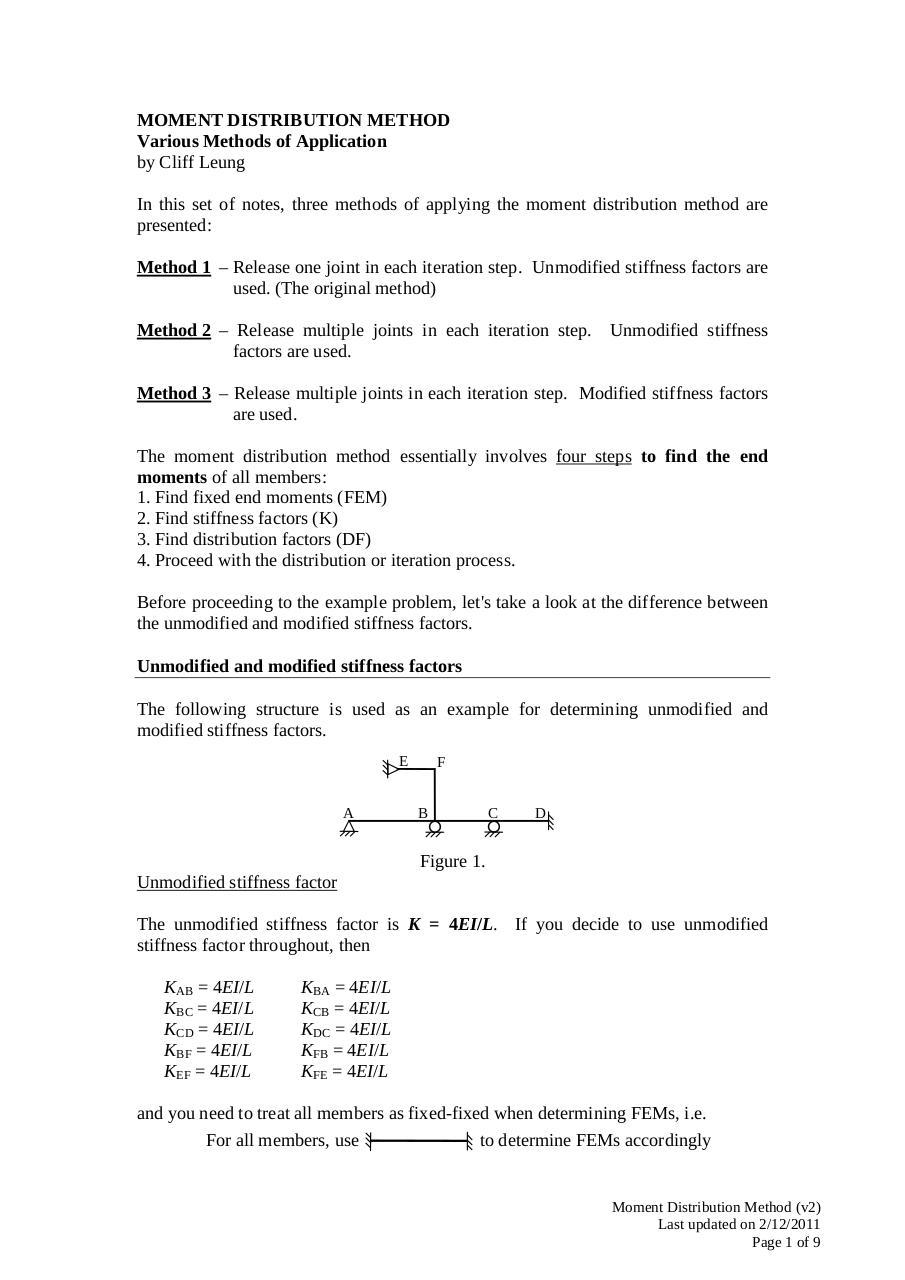# TDII MDM (v2).pdfPage 1 2 3 4 5 6 7 8 9

#### Text preview

MOMENT DISTRIBUTION METHOD
Various Methods of Application
by Cliff Leung
In this set of notes, three methods of applying the moment distribution method are
presented:
Method 1 – Release one joint in each iteration step. Unmodified stiffness factors are
used. (The original method)
Method 2 – Release multiple joints in each iteration step.
factors are used.

Unmodified stiffness

Method 3 – Release multiple joints in each iteration step. Modified stiffness factors
are used.
The moment distribution method essentially involves four steps to find the end
moments of all members:
1. Find fixed end moments (FEM)
2. Find stiffness factors (K)
3. Find distribution factors (DF)
4. Proceed with the distribution or iteration process.
Before proceeding to the example problem, let's take a look at the difference between
the unmodified and modified stiffness factors.
Unmodified and modified stiffness factors
The following structure is used as an example for determining unmodified and
modified stiffness factors.
E

A

F
B

C

D

Figure 1.
Unmodified stiffness factor
The unmodified stiffness factor is K = 4EI/L. If you decide to use unmodified
stiffness factor throughout, then
KAB = 4EI/L
KBC = 4EI/L
KCD = 4EI/L
KBF = 4EI/L
KEF = 4EI/L

KBA = 4EI/L
KCB = 4EI/L
KDC = 4EI/L
KFB = 4EI/L
KFE = 4EI/L

and you need to treat all members as fixed-fixed when determining FEMs, i.e.
For all members, use
to determine FEMs accordingly

Moment Distribution Method (v2)
Last updated on 2/12/2011
Page 1 of 9